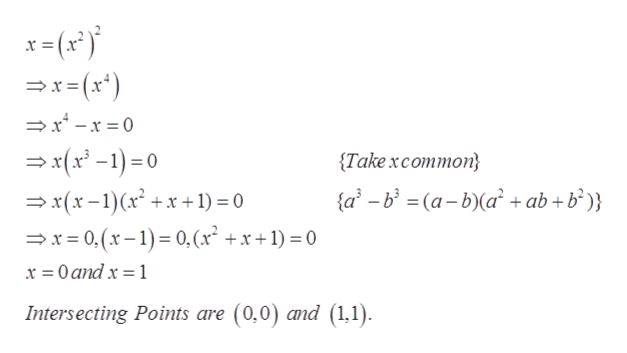# Consider the solid obtained by rotating the region bounded by the given curves about the line x = -6.y=x2, x=y2Find the volume V of this solid.

Question

Consider the solid obtained by rotating the region bounded by the given curves about the line x = -6.

y=x2, x=y2

Find the volume V of this solid.

check_circleExpert Solution
Step 1

Given, function

Step 2

Finding intersection point,help_outlineImage Transcriptionclosex(x*) x -x=0 (x)0 {Take xcommon} {a-b (a-b)(a + ab+b°)} x(x-1)x +x +1) 0 x0,(x-)0, (x2 +x+1)= 0 x 0andx 1 Intersecting Points are (0,0) and (1,1) fullscreen
Step 3

Applying ring ...

### Want to see the full answer?

See Solution

#### Want to see this answer and more?

Solutions are written by subject experts who are available 24/7. Questions are typically answered within 1 hour*

See Solution
*Response times may vary by subject and question
Tagged in

### Integration